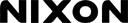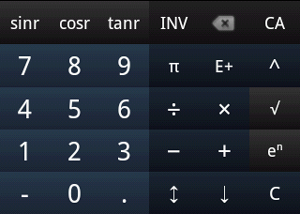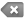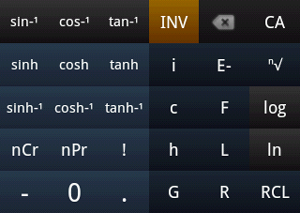# PlottronRPN Calculator

Help and Information > RPN Calculator

## Introduction

RPN Calculator uses Reverse Polish Notation. RPN uses a stack of numbers and does not require parentheses. Functions and operators are evaluated/applied as they are entered into the stack.

To enter number into stack, type number using device or on-screen keyboard. Press [OK] to enter number to stack.

To perform an operation, press operation's button. The function or operator is applied immediately.

Operations are applied to most recent number(s) added into stack. If there are not enough numbers, operation is not performed and no error occurs.

### Examples

• 6+2×3 = ?
 [OK]  [×]  [+] = 12
• sin(π/3+2π*32) = ?
[π]  [÷]  [π]  [×] [×] [+] [sinr] = 0.8660254037844377
• nCr(10,3) = ?
 [OK]  [nCr] = 120
• 3√-27 = ?
 [OK]  [-] [ⁿ√] = -3

## Interface

### ButtonsCA Clear All [CA] Clears every object in the stack. ↕ Swap last two objects in stack.Backspace (for editing current object in stack) ↓ Copy last object in stack. (-) Negate last object in stack. C/OK Clear last object in stack/Add object to stack.i Inserts imaginary unit into stack. c, h, G, F, L, R Insert constant into stack. RCL Recall number. Displays dialog that shows all user-defined variables.

### Evaluation History

The topmost area is the stack. Long-click on any item in the stack to store it as a variable.

### Display Mode

To change display mode, press [MENU] on your device and click [Fractions] or [Complex Numbers]. Then, select display mode from the resulting dialog.

Fraction Modes
Normal Mode Displays numbers as decimals.
Fraction Mode Displays numbers as improper fractions when possible.
Mixed Fraction Mode Displays numbers as mixed fractions when possible.
Complex Modes
Complex Form a+bi Displays complex numbers in the form a+bi.
Complex Form re^Θi Displays complex numbers in polar form, e.g.: 1.0 e0.7853981633974482i

## Statistical Calculations

To show list of statistical calculations for every real number in stack: press [MENU] on device and click Statistics in resulting menu.

#### Single-variable statistics displayed:

• Mean of samples
• Σx Sum of samples
• Σx² Sum of squares of samples
• Sx Standard deviation
• σx Population standard deviation
Click on one of these variables to insert it into stack.

## Variables and Constants

See Variables and Constants in Calculator Help.

• To recall a user-defined variable, press Recall [RCL] key (INV > C/OK), followed by variable in list that appears.
• To store an object into a user-defined variable: long press object in stack, then select variable in resulting menu.

## Preferences

See Preferences in Calculator Help.

## Errors

See Errors in Calculator Help.

If an error occurs during an operation, an error is added to the stack. No more operations may be performed until either the stack or the error is cleared with [CA] or [C].